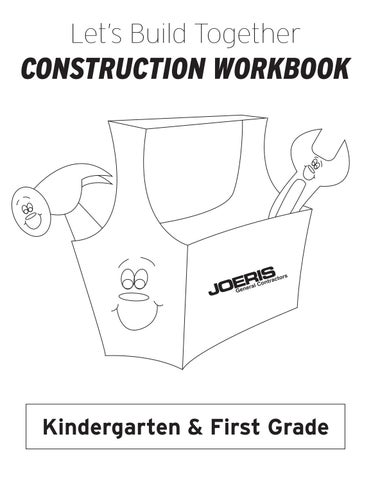Letâ&amp;#x20AC;&amp;#x2122;s Build Together

CONSTRUCTION WORKBOOK

Color the letter!

Color the Râ&amp;#x20AC;&amp;#x2122;s and râ&amp;#x20AC;&amp;#x2122;s red. i

y

o

B

a Z

w G

X

f

R

a Z R

e j T

h

m

g

a p

i n

k K

r

K r

G

z

R

r f

O P

d r

R

E

S

r

K

J

n

H

t

e

W

q

L

u x

U M

H B

A

d y

Copy the word!

Form a sentence!

Kate and the paint Steve house.

2 + 3 = 5 + 2 =

4 + 3 =

Subtraction!

5 - 2 = 7 - 4 =

4 - 2 =

Finish the pattern.

Pick one for each pattern

Circle the picture that is different.

Connect the dots!

19

1

20

2 3

18

4

17 16

5

15

6 7

14 13

8 12

11

Hard Hat

10

9